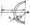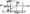# Advanced Mechanics of Solids Dec-2011

UNIT – I

1 (a) Explain body force and surface force.

(b) Define the normal and shear stress components.

(c)  In a triaxial stress system, the six components of the stress at a point are given below :

ctx. = 6MN/m2 xxy = xyx = 1 MN/m2

av = 5MN/m2 T =1=3 MNI m2

az = 4MN/m2 Xzx = Xxz = IMNIm2

Find the magnitude of three principal stresses.

OR

1 (a) What is Lame’s stress ellipsoid ?

(b) Derive the differential equations of equilibrium for 3D state of stress on a body.

(c) A cylindrical boiler, 180 cm in diameter, is made of plates 1.8 cm thick, and is subjected to an internal pressure 1400 Kpa. Determine the maximum shearing stress in the plate at point P and the plane on which it acts.

UNIT – II

(a) Define state of strain at a point. Derive the deformation in the neighbourhood of a point.

(b) The following displacement field is imposed on a bodyu = (xyi 4- 3x2zj + Ak)\ 0~2

Consider a point P and a neighbouring point ‘Q where PQ has the following direction cosines. nx =0.200, ny =0.800, nz =0.555 Point P has coordinates (2,1,3). If PQ = AS, find the components of P’Q’ after deformation.

OR

(a) What are the compatibility conditions ? Explain in detail.

(b) The following state of strain exists at a point P

0.02 -0.04 0 -0.04 0.06 0.02

0 -0.02 0

Determine the principal strains and the directions of the maximum and minimum principal strains.

UNIT – III

3 (a) Derive and explain the generalized Hooke’s law ?

(b) A cubical element is subjected to the following state of stress.

crx = 1 OOMPa, <sy – 20MPa, Gz – -40MPa , xxy = xV2 = Xzx = 0

Assuming the material to be homogeneous* and isotropic, determine the principal shear strains and the octahedral

shear strain, if £ = 2 x 105MPa and v = 0.25.

OR

3 (a) Write short notes on isotropy, anisotropy and orthotropy.

(b) Derive expression for Elastic constant G in terms of Lame’s coefficients for Isotropic material.

(c) Derive stress strain relations for Isotropic material in terms of Lame’s coefficient (X and |0,).

UNIT-IV

4 (a) What do you mean by curved beam ? Explain in detail the difference between symmetrical bending and unsymmetrical bending.

(b) A hook of circular section 25 mm diameter and radius of curvature of its central axis is 25 mm carries a load of 5kN. Calculate the maximum stress in the hook.

OR

4 (a) Define shear centre and shear flow.

(b) Show that the shear centre for the section shown in figure is at measured from point 0.(c)    The figure shows a simply supported beam having span 4m and loaded at an angle 30° to the vertical. The magnitude of load is 2kN. Modulus of elasticity (E) of beam is 210 . GPa. Evaluate the stress at point A and B. Section of the beamUNIT – V

5. (a) Derive the expression for radial and circumferential stresses

(Oy,a0) induced in a thick cylinder which is subjected to internal pressure alone. Draw also stress profiles.

(b) A flat steel turbine disk of 75 cm outside diagram and 15 cm inside diameter rotates at 3000 rpm, at which speed the blades and shrouding cause a tensile rim loading of 4312KPa. The maximum stress at this speed is to be 114072 KPa. Find the maximum shrinkage allowance on the diameter when the disk and the shaft are rotating.

OR

5. (a) Prove that thickness of a disc of uniform strength is given Where tc is the thickness at r = 0

(b) Compute the largest value of ’radial’ and ‘hoop stress’ for a rotating disc of internal diameter 150mm and external diameter 300mm. The disc is rotating at 1500 rpm. for the disc material, density p = 7000 ym\$ and v = 0.3-